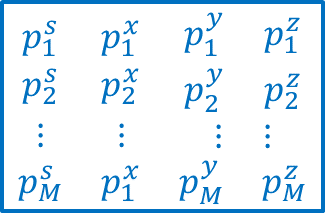# 5.4.5. Model Norm file¶

This file contains the four exponent values within the model used to control the sparsity applied on the model and its gradients [ps px py pz]. The following is the file structure of the model fileEach $$p_{i,j,k}$$ is the norm value in the $$[i,j,k]^{th}$$ model cell $$s$$ denotes the l_p-norm on the model, $$x$$ denotes the l_p-norm on the model gradients along the x-axis (Easting), $$y$$ denotes the $$l_p$$-norm on the model gradients along the y-axis (Northing), $$z$$ denotes the l_p- norm on the model gradients along the z-axis (Vertical). Values must be on the interval $$0 \leq p \leq 2$$. Indices $$[i, j, k]=[1, 1, 1]$$ is defined as the cell at the top, south-west corner of the model. The total number of lines in this file should equal $$NN \times NE \times NZ$$, where $$NN$$ is the number of cells in the north direction, $$NE$$ is the number of cells in the east direction, and $$NZ$$ is the number of cells in the vertical direction. The model ordering is performed first in the z-direction (top-to-bottom), then in the easting, and finally in the northing.